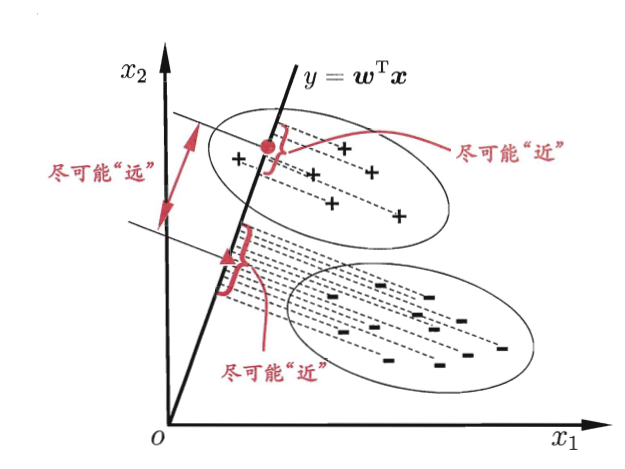# LDA线性判别分析

hit2015spring $\frac {hit2015spring}{晨凫追风}$Xi $X_i$:第 i0,1 $i \in{{0,1}}$类的样本集合
μi $\mu_i$:第 i0,1 $i \in{{0,1}}$类的均值向量
i $\sum_i$:第 i0,1 $i \in{{0,1}}$类的协方差矩阵

1、同类抱团更加紧密
2、不同类分的开

wT0w+wT1w $w^T\sum_0w + w^T\sum_1w$让他们尽可能的小

wTμ0wTμ122 $\|w^T\mu_0 - w^T\mu_1\|_2^2$

J=wTμ0wTμ122wT0w+wT1w $J=\frac{\| w^T\mu_0 - w^T\mu_1\|_2^2}{w^T\sum_0w + w^T\sum_1w}$

Sw=0+1=x1X0(xμ0)(xμ0)T+x1X1(xμ0)(xμ0)T $S_w=\sum_0 + \sum_1=\sum\limits_{x\in1X_0}{(x-\mu_0)(x-\mu_0)^T}+\sum\limits_{x\in1X_1}{(x-\mu_0)(x-\mu_0)^T}$

Sb=(μ0μ1)(μ0μ1)T $S_b=(\mu_0-\mu1)(\mu_0-\mu_1)^T$

J=wTSbwwTSww$J=\frac{w^TS_bw}{w^TS_ww}$

09-26
03-1708-28
12-223万+
07-02
03-04